## VideoPhoto

Showing posts with label Gielis. Show all posts
Showing posts with label Gielis. Show all posts

## 05 August, 2023

### Shapes Based On Johan Gielis's Superformula

The Gielis's Superformula is used to model variety of abstract and natural shapes. It finds application in biology, technology, mathematics, and physics.

I'm providing here a small representation of shapes created with my relatively simple VBA macro listed at the end of this post. You can experiment with different values of the parameters a,b,m,n1,n2, and n3 to get all kinds of shapes and find some useful models. To control the resolution of your shapes you can adjust the number of points, nP. Optionally, I've applied some Excel formatting of the plots.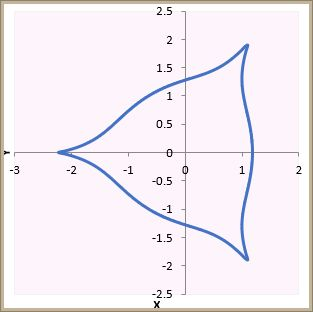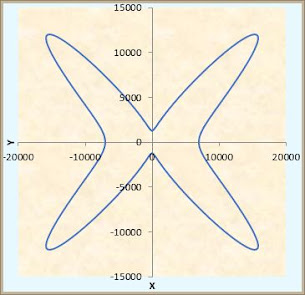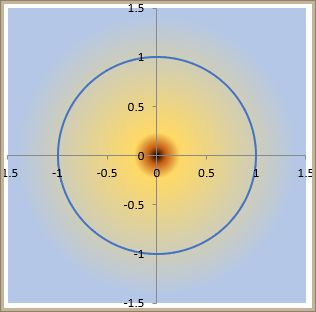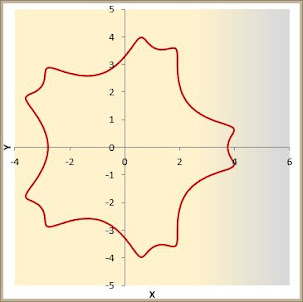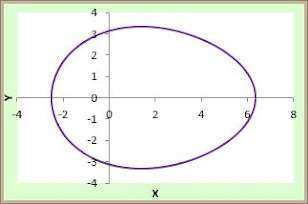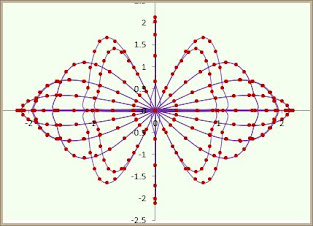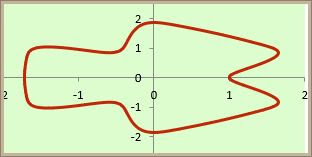Sub SuperformulaPlot()
'Generates Gielis's Superformula plot
'Calculates X and Y values and assigns them to the chart series
'Creates a 2D scatter plot; no markers

Dim nP As Integer, i As Integer
Dim xVal() As Double, yVal() As Double, theta As Double, r As Double
Dim a As Double, b As Double, m As Double, n1 As Double, n2 As Double, n3 As Double
Dim chartObj As ChartObject
nP = 500    'You can adjust the number of points to increase/decrease the resolution of the curve
a = WorksheetFunction.RandBetween(1, 5) 'Random parameters a,b,m,n1,n2,n3; experiment!
b = WorksheetFunction.RandBetween(1, 5)
m = WorksheetFunction.RandBetween(1, 10)
n1 = WorksheetFunction.RandBetween(1, 10)
n2 = WorksheetFunction.RandBetween(1, 20)
n3 = WorksheetFunction.RandBetween(1, 10)
'a = 1: b = 1: m = 2: n1 = 5: n2 = 5: n3 = 5    'just some default values
ReDim xVal(1 To nP)
ReDim yVal(1 To nP)
For i = 1 To nP
theta = 2 * WorksheetFunction.Pi * (i - 1) / nP
r = (Abs(Cos(m * theta / 4) / a) ^ n2 + Abs(Sin(m * theta / 4) / b) ^ n3) ^ (-1 / n1)
xVal(i) = r * Cos(theta)
yVal(i) = r * Sin(theta)
Next i
'Create a 2D scatter plot
Set chartObj = ActiveSheet.ChartObjects.Add(Left:=120, Width:=500, Top:=10, Height:=400)
With chartObj.Chart
.ChartType = xlXYScatterSmoothNoMarkers
.SeriesCollection.NewSeries
.SeriesCollection(1).Values = yVal      'Add data to the chart
.SeriesCollection(1).xValues = xVal
.HasLegend = False
.HasTitle = True
.ChartTitle.Text = "SuperPlot "& a & "-"& b & "-"& m & "-"& n1 & "-"& n2 & "-" & n3 & "; "& nP
.Axes(xlCategory, xlPrimary).HasTitle = True
.Axes(xlCategory, xlPrimary).AxisTitle.Text = "X"
.Axes(xlValue, xlPrimary).HasTitle = True
.Axes(xlValue, xlPrimary).AxisTitle.Text = "Y"
End With
End Sub